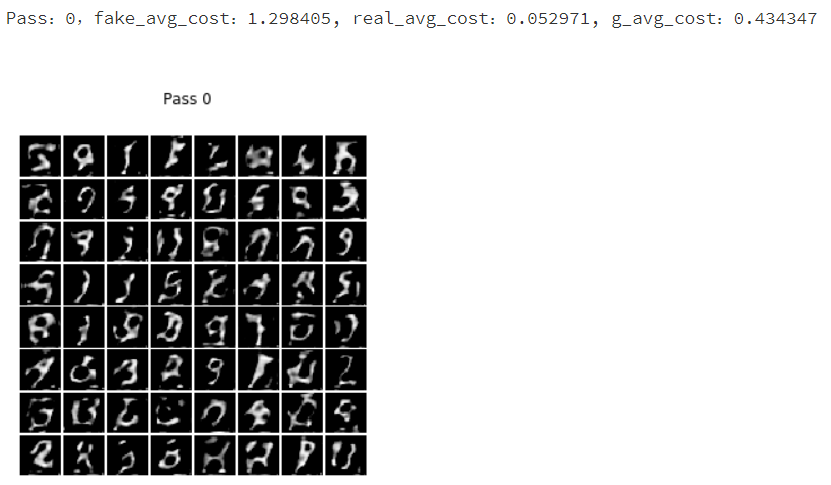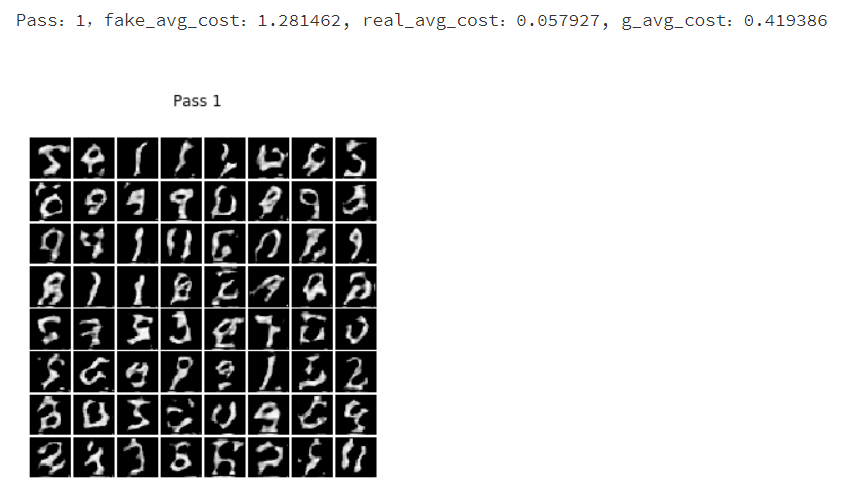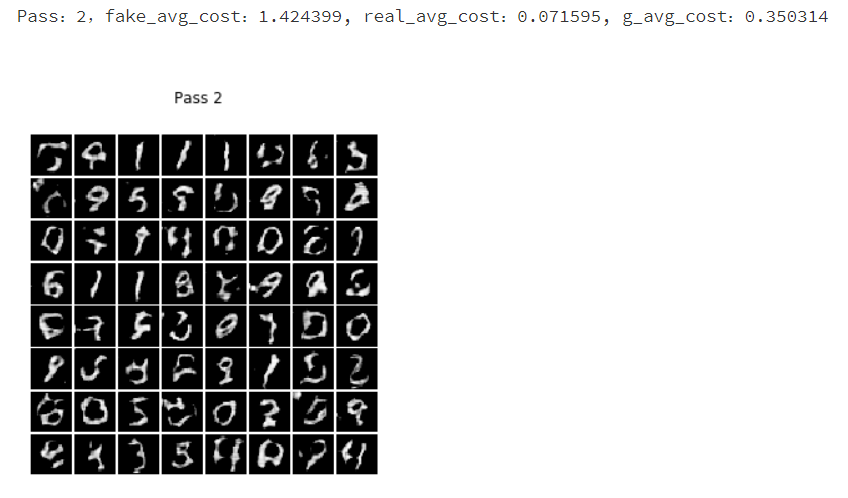# 训练并预测

``````import numpy as np
import matplotlib.pyplot as plt
``````

## 定义网络

``````# 定义生成器
def Generator(y, name="G"):
def deconv(x, num_filters, filter_size=5, stride=2, dilation=1, padding=2, output_size=None, act=None):
return fluid.layers.conv2d_transpose(input=x,
num_filters=num_filters,
output_size=output_size,
filter_size=filter_size,
stride=stride,
dilation=dilation,
act=act)
with fluid.unique_name.guard(name + "/"):
# 第一组全连接和BN层
y = fluid.layers.fc(y, size=2048)
y = fluid.layers.batch_norm(y)
# 第二组全连接和BN层
y = fluid.layers.fc(y, size=128 * 7 * 7)
y = fluid.layers.batch_norm(y)
# 进行形状变换
y = fluid.layers.reshape(y, shape=(-1, 128, 7, 7))
# 第一组转置卷积运算
y = deconv(x=y, num_filters=128, act='relu', output_size=[14, 14])
# 第二组转置卷积运算
y = deconv(x=y, num_filters=1, act='tanh', output_size=[28, 28])
return y
``````

``````# 判别器 Discriminator
def Discriminator(images, name="D"):
# 定义一个卷积池化组
def conv_pool(input, num_filters, act=None):
return fluid.nets.simple_img_conv_pool(input=input,
filter_size=5,
num_filters=num_filters,
pool_size=2,
pool_stride=2,
act=act)

with fluid.unique_name.guard(name + "/"):
y = fluid.layers.reshape(x=images, shape=[-1, 1, 28, 28])
# 第一个卷积池化组
y = conv_pool(input=y, num_filters=64, act='leaky_relu')
# 第一个卷积池化加回归层
y = conv_pool(input=y, num_filters=128)
y = fluid.layers.batch_norm(input=y, act='leaky_relu')
# 第二个卷积池化加回归层
y = fluid.layers.fc(input=y, size=1024)
y = fluid.layers.batch_norm(input=y, act='leaky_relu')
# 最后一个分类器输出
y = fluid.layers.fc(input=y, size=1, act='sigmoid')
return y
``````

## 定义训练程序

``````# 创建判别器D识别生成器G生成的假图片程序
train_d_fake = fluid.Program()
# 创建判别器D识别真实图片程序
train_d_real = fluid.Program()
# 创建生成器G生成符合判别器D的程序
train_g = fluid.Program()
# 创建共同的一个初始化的程序
startup = fluid.Program()
# 噪声维度
z_dim = 100
``````

``````# 从Program获取prefix开头的参数名字
def get_params(program, prefix):
all_params = program.global_block().all_parameters()
return [t.name for t in all_params if t.name.startswith(prefix)]
``````

``````# 训练判别器D识别真实图片
with fluid.program_guard(train_d_real, startup):
# 创建读取真实数据集图片的data，并且label为1
real_image = fluid.layers.data('image', shape=[1, 28, 28])
ones = fluid.layers.fill_constant_batch_size_like(real_image, shape=[-1, 1], dtype='float32', value=1)

# 判别器D判断真实图片的概率
p_real = Discriminator(real_image)
# 获取损失函数
real_cost = fluid.layers.sigmoid_cross_entropy_with_logits(p_real, ones)
real_avg_cost = fluid.layers.mean(real_cost)

# 获取判别器D的参数
d_params = get_params(train_d_real, "D")

# 创建优化方法
optimizer.minimize(real_avg_cost, parameter_list=d_params)
``````

``````# 训练判别器D识别生成器G生成的图片为假图片
with fluid.program_guard(train_d_fake, startup):
# 利用创建假的图片data，并且label为0
z = fluid.layers.data(name='z', shape=[z_dim, 1, 1])
zeros = fluid.layers.fill_constant_batch_size_like(z, shape=[-1, 1], dtype='float32', value=0)

# 判别器D判断假图片的概率
p_fake = Discriminator(Generator(z))

# 获取损失函数
fake_cost = fluid.layers.sigmoid_cross_entropy_with_logits(p_fake, zeros)
fake_avg_cost = fluid.layers.mean(fake_cost)

# 获取判别器D的参数
d_params = get_params(train_d_fake, "D")

# 创建优化方法
optimizer.minimize(fake_avg_cost, parameter_list=d_params)
``````

``````# 训练生成器G生成符合判别器D标准的假图片
with fluid.program_guard(train_g, startup):
# 噪声生成图片为真实图片的概率，Label为1
z = fluid.layers.data(name='z', shape=[z_dim, 1, 1])
ones = fluid.layers.fill_constant_batch_size_like(z, shape=[-1, 1], dtype='float32', value=1)

# 生成图片
fake = Generator(z)
# 克隆预测程序
infer_program = train_g.clone(for_test=True)

# 生成符合判别器的假图片
p = Discriminator(fake)

# 获取损失函数
g_cost = fluid.layers.sigmoid_cross_entropy_with_logits(p, ones)
g_avg_cost = fluid.layers.mean(g_cost)

# 获取G的参数
g_params = get_params(train_g, "G")

# 只训练G
optimizer.minimize(g_avg_cost, parameter_list=g_params)
``````

## 训练并预测

``````# 噪声生成
while True:
yield np.random.normal(0.0, 1.0, (z_dim, 1, 1)).astype('float32')
``````

``````# 读取MNIST数据集，不使用label
def r():
yield img.reshape(1, 28, 28)
return r
``````

``````# 显示图片
def show_image_grid(images, pass_id=None):
# fig = plt.figure(figsize=(5, 5))
# fig.suptitle("Pass {}".format(pass_id))
# gs = plt.GridSpec(8, 8)
# gs.update(wspace=0.05, hspace=0.05)

for i, image in enumerate(images[:64]):
# 保存生成的图片
plt.imsave("image/test_%d.png" % i, image)
# 以下代码在jupyter可用
#     ax = plt.subplot(gs[i])
#     plt.axis('off')
#     ax.set_xticklabels([])
#     ax.set_yticklabels([])
#     ax.set_aspect('equal')
#     plt.imshow(image, cmap='Greys_r')
# plt.show()

``````

``````# 生成真实图片reader
``````

``````# 创建执行器
# place = fluid.CPUPlace()
place = fluid.CUDAPlace(0)
exe = fluid.Executor(place)
# 初始化参数
exe.run(startup)
``````

``````# 测试噪声数据
test_z = np.array(next(z_generator))
``````

``````# 开始训练
for pass_id in range(5):
for i, real_image in enumerate(mnist_generator()):
# 训练判别器D识别生成器G生成的假图片
r_fake = exe.run(program=train_d_fake,
fetch_list=[fake_avg_cost],
feed={'z': np.array(next(z_generator))})

# 训练判别器D识别真实图片
r_real = exe.run(program=train_d_real,
fetch_list=[real_avg_cost],
feed={'image': np.array(real_image)})

# 训练生成器G生成符合判别器D标准的假图片
r_g = exe.run(program=train_g,
fetch_list=[g_avg_cost],
feed={'z': np.array(next(z_generator))})
print("Pass：%d，fake_avg_cost：%f, real_avg_cost：%f, g_avg_cost：%f" % (pass_id, r_fake, r_real, r_g))

# 测试生成的图片
r_i = exe.run(program=infer_program,
fetch_list=[fake],
feed={'z': test_z})

# 显示生成的图片
show_image_grid(r_i, pass_id)
``````